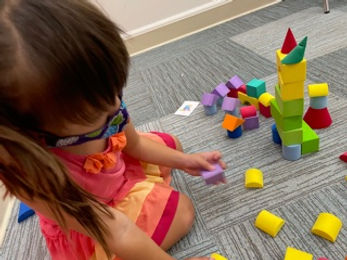## Ms. Kayla

### Target 1​

###### Lesson Type:

New

Number Operation

:

Number System

Understand that numbers have an order (limited to numbers 0 to 50).

###### 1:

Understand that the last number counted depicts the quantity of the objects.

###### 2:

Understand that the number sequence does not change, even when some numbers are missing from a given sequence.

###### 3:

Identify when a given sequence is a correct count sequence.

Pre-K

###### Vocabulary:

Number, Order, First, After, Next, Count

Activities:

1. Students identified numbers and showed the number with pegs. Students counted the pegs once the number was represented. Students, with guidance, placed the number pegs in order from 1-10.

2. When the numbers were in order, student practiced saying the numbers forward and backward.### Home Exploration

###### Guiding Questions:## Absent Students:

### Target 2

:

###### 1:

Use a 2D diagram as a guide or map to build something 3D.

###### 2:

Use directional language to help describe how a 3D and 2D representations are the same (in the picture the red block is in front of the blue block).

Pre-K

###### Vocabulary:

Shapes, Rectangle, Square, Circle, Bridge, Triangle

Activities:

1. Students used building blocks to build 3D structures from images on cards. Students used the image cards as directions on how to build the 3D structures. Students selected the blocks needed to build the structure. Students identified the blocks based on the shape faces. Students were shown how to create shapes by combining two smaller ones. For example, two triangle blocks can be put together to create a cube.### Home Exploration

###### Guiding Questions:### Target 3

:

###### 1:

Classify concrete objects by a single criterion (color, shape, or size).

###### 2:

When given a group of objects, identify the common property and then add to the group with objects that fit the same category.

Pre-K

###### Vocabulary:

Sort, Color, Count,

Activities:

1. Students used farm animals to practice sorting. Students sorted the animals by type and then counted the quantity of each animal. Students counted to about 15-20 animals.### Home Exploration